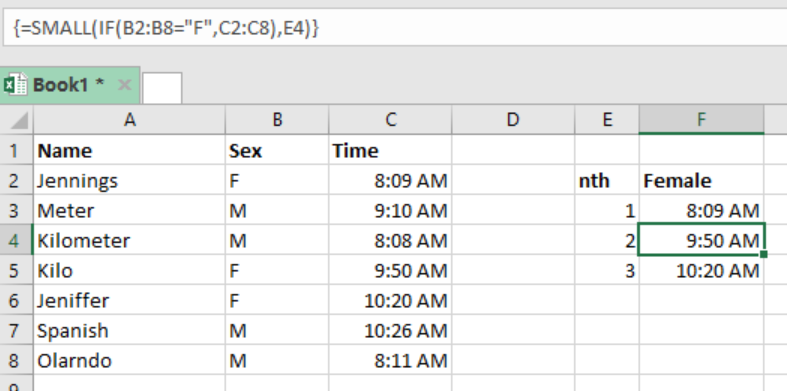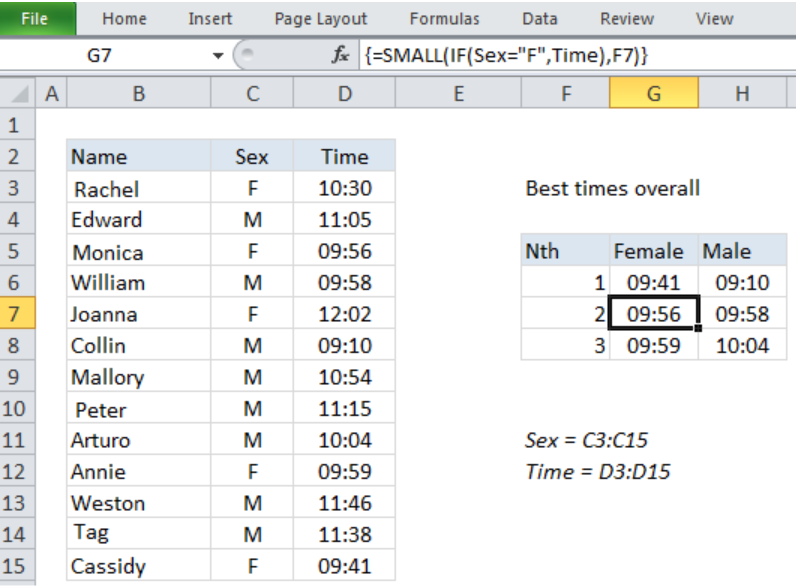Get instant live expert help with Excel or Google Sheets“My Excelchat expert helped me in less than 20 minutes, saving me what would have been 5 hours of work!”

#### Post your problem and you’ll get Expert help in seconds.

Your message must be at least 40 characters
Our professional Expert are available now. Your privacy is guaranteed.

# nth smallest value with criteria

We can use a particular criteria to get the nth smallest value in excel. What we are required to do is provide our particular criteria and then use an array formula that is based on SMALL and IF functions. This post provides a clear guide on how to get the nth smallest value with criteria in excel.Figure 1: Finding nth smallest value in excel

## General formula

`{=SMALL(IF(criteria, values),n)}`

## Understanding the formula

We can use the above formula to get the 1st, 2nd, 3rd smallest value based on a specified criteria.

## How the formula works

• It is easy to work with the SMALL function as it is an automatic function.
• What you are required to do is to supply a range and integer for the nth value to specify its rank.
• When working with this function, we try to disallow it to work on every value in the supplied range. This is why we have to supply a criteria, either Male or Female.
• To make specify either F or M. we use the IF function which provides logical test.
• This is an array formula, and therefore needs to be entered in an array format.

## ExampleFigure 2: Example of how to get nth smallest value with criteria

In this example, we want to find the 2nd and 3rd smallest values in the table. To do this, we proceed as follows;

Step 1: Fill the table with your data.

Step 2: Specify where you want your results to appear. In our example, this is range F5:G8

Step 3: Make cell G7 active and enter the array formula as `{=SMALL(IF(C3:C15= “F”,D3:D15), F7)}`

Step 4: Press Control + Shift + Enter given that this is an array formula.

## Instant Connection to an Expert through our Excelchat Service

Most of the time, the problem you will need to solve will be more complex than a simple application of a formula or function. If you want to save hours of research and frustration, try our live Excelchat service! Our Excel Experts are available 24/7 to answer any Excel question you may have. We guarantee a connection within 30 seconds and a customized solution within 20 minutes.

### Did this post not answer your question? Get a solution from connecting with the expert.Another blog reader asked this question today on Excelchat:
Solution exampleshello i need help creating a formula, if a value does not exsits put 0, if a value exist do nothing
Solved by M. F. in 9 minsCompute the weighted average of students score from a spreadsheet using different weight for each category of marks
Solved by G. E. in 20 minshi there, im making a spread sheet to track progress in a game and would like to create a drop log sheet, i was hoping to use the if function to detect weather a item is logged on a sheet then send the sheet number to a list if so
Solved by K. F. in 60 minsI have a schedule I'm doing with formulas to add workdays (across rows) to a start date column. I would like to write the equation like this: =ifError((workday(b2,1) = cell above, [value of cell above + 1] Help me form this correctly to get the (value of target cell) = (1 more than the value from the cell above) IF the target cell value started equal to the cell above.
Solved by M. J. in 20 minsI need a formula that will subtract the sum of one cell if the sum of another cell is greater than zero, and have it applied to cells across a document that is 30,000 entries and only in certain spots
Solved by F. J. in 20 mins## Subscribe to Excelchat.coAnother blog reader asked this question today on Excelchat: